# Numerical Thermal Analysis of an Electric Oven for Neapolitan Pizzas

Numerical Thermal Analysis of an Electric Oven for Neapolitan Pizzas

Mena CiarmielloBiagio Morrone

Department of Industrial and Information Technology Engineering, Second University of Naples, via Roma 29, 81031 Aversa (CE), Italy

Corresponding Author Email:
mena.ciarmiello@unina2.it
Page:
S351-S358
|
DOI:
https://doi.org/10.18280/ijht.34S223
|
Accepted:
|
Published:
31 October 2016
| Citation

OPEN ACCESS

Abstract:

Neapolitan pizza is traditionally cooked in wood-fired ovens ensuring high quality in cooking pizza and providing an excellence in Italian cooking tradition.

Besides safeguarding Italian cooking tradition, in recent years there is a growing attention on wood-fired oven impacts both on indoor and outdoor air quality and pollution. Thus, optimal design of electric ovens alternative to wood-fired ones is an interesting concern in order to achieve both high quality of Neapolitan pizza cooking and air pollution prevention.

This paper deals with a numerical investigation on thermal conditions provided by an innovative electric oven for Neapolitan pizzas cooking. A three-dimensional numerical model has been developed based on a finite volume scheme to simulate steady and unsteady operating conditions. Radiation effects of inner walls have been considered and the boundary effects of the oven, both radiative and convective, have been carefully modelled.

Results have been examined in terms of heat flux distributions, temperature and velocity fields, showing good cooking conditions for pizzas.

Keywords:

Computational fluid dynamic, Electric oven, Numerical simulation, Radiative heat flux.

1. Introduction

Neapolitan Pizza has its origins at the beginnings of 1700's in Naples, Southern Italy and until the early twentieth century it was a typical Neapolitan meal. Then many commercial pizzerias opened across Italy and abroad and nowadays Neapolitan pizza is one of the most popular worldwide excellence in Italian cooking tradition, being recognized as Traditional Specialty Guaranteed (TSG) by Commission Regulation (EU) n. 97/2010 and requested for UNESCO recognition.

Neapolitan Pizza is made based on the specification of production published by , having a diameter not greater than 35 cm and a thickness of the dough disk not larger than 0.4 cm and 1-2 cm at its center and crust edge, respectively.

Furthermore Neapolitan Pizza is traditionally cooked in wood-fired ovens, at a temperature of the dome and bed of 430°C and 485°C, respectively and with a cooking time never exceeding 90 s , guaranteeing a product of excellence in Italian cooking tradition.

In recent years there has been a growing interest on wood-fired ovens impacts due to stack emissions of wood combustion.

Although data concerning stack emission from a typical wood-fired oven for pizzas are very scarce, it is widely recognized that combustion of biomasses, such as wood, may lead to the release of many airborne pollutants, including particulate matter, occurring significant impact both on air quality and human health exposure.

Fine particles and hazard air pollutants, such as polycylic aromatic hydrocarbons (PAHs), dioxins and furans are generally products of combustion, depending on completeness of combustion  and ninety organic group of compounds in emissions from wood combustion have been identified , depending on several parameters, such as operating conditions, firewood and burn-off temperature. At low burn-off temperatures (<500°C), aromatic compound  and polychlorinated dioxins and furans are formed in presence of chlorine , while at high burn-off temperatures (<1200°C), NOx can form from atmospheric nitrogen .

Referring to indoor air impacts, effects of wood-burning fireplaces operated with mature wood were investigated, finding levels of CO, CO2, NO, NO2, TVOC, formaldehyde and acetaldehyde not exceeding from the German Federal Environment Agency both from short and long-term, while elevated concentrations of ultra fine particles (UFPs) and benzene were detected . Furthermore polyaromatic hydrocarbons (PAHs) emissions are relevant for small-scale combustor, such as wood stoves and domestic central heating boilers .

As far as particle emissions, particle matter distribution and concentrations were measured in 15 pizzerias, finding an increase in exposure during wood burning operation compared to background levels, with further increase during the cooking of pizzas .

For this reason, optimal design of electric ovens alternative to wood-fired ones is a topic of great interest in order to achieve high quality of Neapolitan pizza cooking and air pollution prevention and protection of health.

To the authors’ knowledge few numerical models have been developed to simulate electrical ovens. In  the authors dealt with numerical simulation of the flow field in an isothermal airflow in an oven for heating food using a complex numerical model for turbulence, since their main interest was on the flow field. The modelling of transient natural convection in oven for food was investigated in , further the numerical model employed in  studied both heat transfer and flow field in a pilot oven for bakery. Further, a closed cavity with partially heated wall was reported in  and turbulent mixed convection in a ventilated enclosure was investigated in .

This paper deals with a numerical investigation on thermal conditions provided by an innovative electric oven for Neapolitan pizzas cooking. A three-dimensional numerical model has been developed based on a finite volume scheme approach to simulate steady and unsteady cooking conditions. The inner walls of the cooking chamber are made of solid refractory bricks, with an adjacent layer of rock wool (Figure 1).

## figure_1.pngFigure 1. Sketch of the investigated domain

Thermal conditions into the cooking chamber are provided by electrical resistances, embedded into the upper and bottom layer of the bricks, respectively.

In addition, great care has been devoted to the radiation effects of inner walls and the boundary effects of the oven, both radiative and convective, have been carefully modeled, since they can seriously affect the results.

Results are presented in terms of temperature, heat flux distributions, and velocity fields to assess the validity of such type of oven for cooking Neapolitan pizzas correctly.

2. Mathematical Model

The oven is represented as an enclosure whose air is heated by electrical resistances embedded in solid refractory bricks. The enclosure is limited by solid walls which act as boundaries and insulation layers.

The mathematical model has been developed by considering all the heat transfer mechanisms which occur in the investigated domain. The domain has been decomposed mainly in two subdomains: the solid one, which represents the shell of the structure with the electrical resistances, and the cooking chamber where the pizzas are placed to be cooked, as the cross section view shown in Figure 1.

The solid subdomain walls are made of clay refractory bricks and mineral rock wool, which acts as insulating material for the oven. The thickness of the refractory bricks is equal to 5.0 cm, whereas the rock wool is 15.0 cm thick.

The corresponding thermo-physical properties have been obtained by brick manufacturers and are reported in Table 1. The materials have been assumed to be isotropic, and the thermal conductivity of refractory bricks considered as a function of the temperature.

Table 1. Main thermo-physical properties of the solid materials

 Material λ [W/(m K)] ρ [kg/m3] c [J/(kg K)] Refractory bricks 0.770 @ 293 K 1,850 1,050 Rock wool 0.04 50 840

The electrical resistances, made of nickel alloy, are embedded in the solid structure on the upper and lower walls of the oven. The two resistances with electrical power of 11.2 kW and 3.4 kW, upper and lower ones respectively, have been implemented to mimic a function that allows an on/off cycle with a period set up by the user. After the heating period, when the oven is ready for cooking pizzas, the resistances are not on for the entire period, but they undergo an on/off cycle by a temperature controller.

The cooking chamber is the subdomain where the disks representing the pizzas are placed and cooked.

The air inside the cooking chamber has been considered as a perfectly transparent medium so that the radiative heat transfer takes place only among the inner-side walls of the enclosure.

The continuity, momentum and energy equations for three-dimensional coordinate system in the different investigated subdomains, can be read as:

• continuity equation

$\frac{\partial \rho}{\partial t}+\nabla \cdot(\rho \overline{V})=0$(1)

• momentum equations (using the summation convention and the substantive derivative) 

\begin{aligned} \rho \frac{D V_{i}}{D t}=&-\frac{\partial p}{\partial x_{i}}+\frac{\partial}{\partial x_{j}}\left[\mu\left(\frac{\partial V_{i}}{\partial x_{j}}+\frac{\partial V_{j}}{\partial x_{i}}\right)\right]-\rho_{0} \overline{g} \beta\left(t-T_{0}\right)+\\ &-\frac{\partial}{\partial x_{j}}\left(-\rho \overline{V_{i}^{\prime} V_{j}^{\prime}}\right) \end{aligned}(2)

• energy equation in the fluid domain

$\frac{\partial}{\partial t}\left(\rho c_{p} T\right)+\nabla \cdot\left[\overline{V}\left(\rho c_{P} T\right)\right]=\nabla\left(\lambda_{e f f} \nabla T\right)$(3)

• energy equation in the solid domain with generation

$\rho c \frac{\partial T}{\partial t}=\nabla(\lambda \nabla T)+e_{g e n}$(4)

being ρcp the thermal inertia of the system, l the thermal conductivity and egen the thermal generation per unit volume which takes place in the solid domain.

In Eq. the body force term uses the Boussinesq approximation, considering the density constant and equal to a reference value and its variation related to the temperature difference, being b the coefficient of thermal expansion and g the gravity vector. Eqs - are supplemented with the k-e equations to consider standard turbulent model for the closure of the momentum equations, which are required to describe the turbulent stress, last term of Eq., and the diffusivity leff in Eq. , which is the total thermal diffusivity, including the turbulent term.

Radiative heat transfer is accounted only among solid surfaces of the cooking chamber by means of the surface-to-surface method, thus considering the fluid medium, air, as perfectly transparent. The surfaces are assumed to be gray and diffuse. The local radiative heat flux is evaluated at the inner solid surfaces as:

$q_{i}\left(\mathbf{r}_{\mathbf{i}}\right)=J_{i}\left(\mathbf{r}_{\mathbf{i}}\right)-G_{i}\left(\mathbf{r}_{\mathbf{i}}\right)$(5)

and after some manipulations, as shown in  the radiative balance equation reads, at each elemental surface i:

\begin{aligned} \frac{q_{i}\left(\mathbf{r}_{i}\right)}{\varepsilon_{i}}=&+\sum_{j} \frac{1-\varepsilon_{j}}{\varepsilon_{j}} \int_{A_{j}} q_{j}\left(\mathbf{r}_{j}\right) d F_{d i-d j}\left(\mathbf{r}_{j}, \mathbf{r}_{i}\right)+\\ &-\sum_{j} \int_{A_{j}} \sigma\left[T_{i}^{4}\left(\mathbf{r}_{i}\right)-T_{j}^{4}\left(\mathbf{r}_{j}\right)\right] d F_{d i-d j}\left(\mathbf{r}_{j}, \mathbf{r}_{i}\right) \end{aligned}(6)

The wall emissivity has been assumed equal to 0.9 for all the surfaces facing the cooking chamber, a typical value for bricks.

3. Numerical Model

The nonlinear partial differential equations are solved using the Finite Volume Method (FVM) for transient regime. The numerical model used to solve the discretized differential equations is the SIMPLER for the velocity-pressure coupling with a first order upwind discretization for the convective terms. The under-relaxation parameters for pressure and momentum equations are set to 0.3 and 0.7, respectively. The transient regime is solved using an implicit First Order formulation for the time advancement. The domain is meshed using hexahedral elements which cover the different domains with different mesh sizes. Different cell numbers have been tested in the calculations as displayed in Table 2.

The configuration factors among the surfaces involved in the radiative heat transfer have been evaluated using the following relation, for each elemental area di:

$F_{d i \rightarrow j}\left(r_{i}\right)=\int_{A_{j}} d F_{d i \rightarrow d j}\left(r_{i}, r_{j}\right)=\int_{A_{j}} K\left(r_{i}, r_{j}\right) d A_{j}$(7)

being K(ri, rj) the kernel of the integral equation, defined as:

Table 2. Data results averaged over the bottom surface as a function of the cells number

 Case Cells Total Heat Flux (W/m-2) Radiative Heat Flux (W/m-2) Nu 1 35,718 275.4 238.9 24.4 2 93,227 256.1 183.0 17.8 3 671,128 239.3 145.5 16.6

$K\left(\mathbf{r}_{i}, \mathbf{r}_{j}\right)=\frac{d F_{d i \rightarrow d j}\left(\mathbf{r}_{i}, \mathbf{r}_{j}\right)}{d A_{j}}$(8)

The boundary conditions imposed on external walls are based on a mixed convective and radiative heat flux, this latter defined as qrad = εσ(Tw4- Tp4), being ε the emissivity, σ the Stefan-Boltzmann constant, Tp the external temperature of the surrounding enclosure and Tw the local temperature of the wall shell. The open section has been modeled as an open boundary with an assigned discharge coefficient and a specified external boundary temperature, to allow airflow flowing into or out of the cooking chamber.

3.1 Numerical validation

In order to assess the quality of the employed discretization grid, three different meshes have been compared in terms of total, radiative heat flux and Nusselt number averaged over the bottom wall of the cooking chamber. As it can be seen from Table 2, the variation of the average quantities is large from Case 1 and 3, Case 1 being the mesh with about 36,000 cells employed and Case 3 with about 670,000 cells, i.e. about twenty times more cells than the previous mesh. Considering the surface average heat flux quantity calculated at steady state for the bottom surface of the cooking chamber, the overestimation of Case 1 is about +15% compared with the value for Case 3. Even worse the comparison for the radiative heat flux, with a discrepancy larger than 60%. This is due to the difficulty in dealing correctly with the configuration factors, when the number of involved faces is low. A similar situation occurs for the Nusselt number with an overestimation of about 45%.

Comparing Case 2 and 3, smaller overestimations are observed for the three compared quantities, anyway being larger than 20% for the radiation heat flux. Thus results are presented in the next for the Case 3, also because the radiation represents the most important mechanism of heat transfer in the cooking chamber and cannot be wrongly evaluated.

4. Results and Discussion

Starting with the oven at room temperature, assumed equal to 298 K, the electrical resistances are on. The heating period lasts several hours. In the subsequent condition the resistances experience the on/off cycle with a reference average temperature set to 758 K and different periods. When the average temperature attains a reference value, equal to 758 K, the resistances experience the on/off cycle with different periods.

Temperature, heat  flux  and  velocity  distribution  in the cooking chamber are reported in the next at two different times, one during the heating phase, at t = 1.3 h, the other once a steady-state condition is attained, at about t = 4.5 h.

The temperature distributions for the heating phase are displayed in Figure 2, at the bottom (Figure 2 a) and upper walls (Figure 2 b) of the cooking chamber, respectively. The two distributions look like quite similar when the oven is during the heating phase. Anyway, some differences are observed. The largest temperatures are attained on the upper surface with a maximum value of about 840 K the lowest being about 730 K. A similar situation occurs at the bottom surface with a maximum around 820 K and a minimum temperature value of 655 K, the lowest values attained near the air flow entrance from the open section.

## figure_2a.png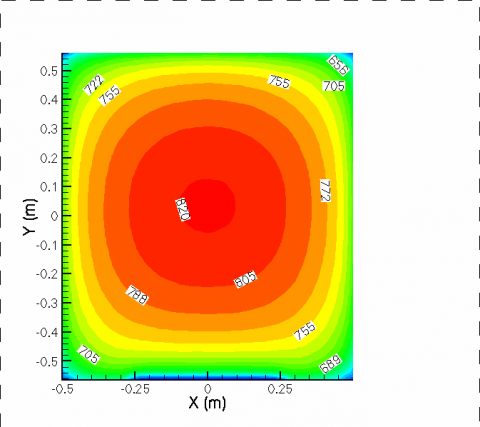(a) Bottom surface of the cooking chamber

## figure_2b.png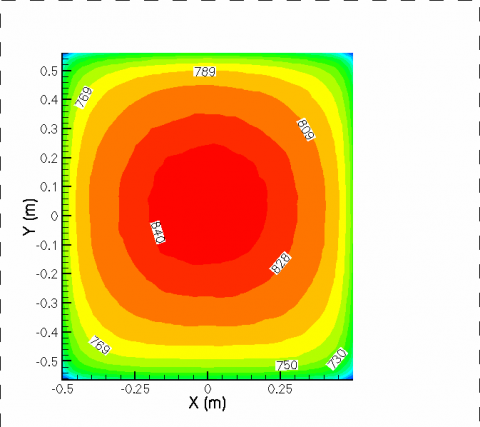(b) Upper surface of the cooking chamber

Figure 2. Temperature distribution at time t = 1.3 h

In addition, large tangential thermal gradients are observed on the bottom surface, whereas smaller gradients are displayed on the upper surface. Different temperature distributions between upper and lower surfaces bring large radiative heat transfer between the two surfaces. In this phase the different temperature values are due to the lower electrical power at the bottom resistance.

These observations are supported by Figure 4, where the temperature is reported as a function of the Y-coordinate at three locations both on the upper and bottom surfaces. The three lines a, b, c are drawn parallel to the Y-coordinate and are located at X =0.0 (line a), -0.2 (line b) and -0.4 m (line c), as shown in Figure 3.

## figure_3.png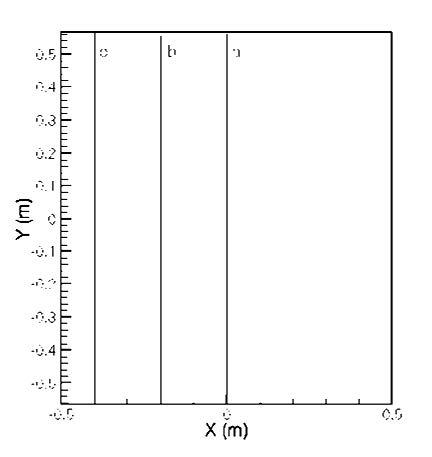Figure 3. Location of lines a, b, c used to plot the temperature profiles (see Figure 4)

## figure_4.png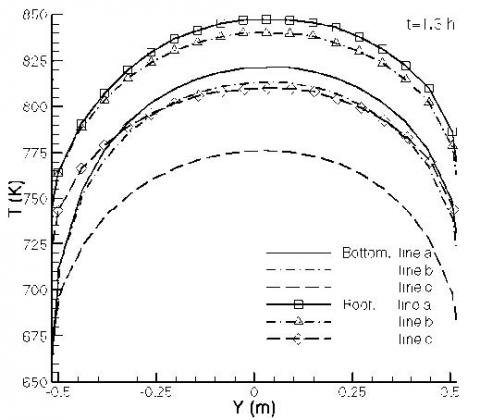Figure 4. Temperature as a function of the Y coordinate

Temperatures show higher values on the upper surface, as already observed, with nearly a parabolic profile. Similar distributions are observed for the bottom surface with lower values. At the open inlet surface, Y = -0,5 m, temperatures are much lower on the bottom surface, attaining about 670 K because the fresh air suctioned into the cooking chamber is at environment temperature, thus cooling that part of the wall. Considering the corresponding coordinates of the upper and bottom surface there is an average temperature difference of about 20 K.

After 4.5 h from the start of heating of the oven, steady state is attained and temperature distributions over the bottom, and upper surfaces, Figure 5, are observed and they display different from the previous ones. In fact, Figure 5 a shows the attainment of a nearly uniform temperature distribution in the inner part of bottom surface, with the highest value equal to 757 K. The zones close to the vertical walls show nearly the same temperature of the inner part, thanks to the rock wool thickness which insulates very well the cooking chamber.

Figure 5 b shows the temperature field on the upper surface. A larger zone compared with the bottom surface shows high temperatures, due to the larger power electrical supply resistance embedded in the refractory bricks and because the air flowing from outside has been already heated by the bottom surface.

The inner part shows value of about 756 K and the region near the open section shows the lowest temperatures, which are larger than those on the bottom surface. Nearly uniform temperature profiles are observed in Figure 6. When the steady state is reached (t = 4.5 h), temperature distribution is more uniform and this type of profiles are attained both at the bottom and upper plates. This results in very good cooking condition for pizzas. Further, the total heat flux exchanged between the bottom surface and the other surrounding surfaces for the heating condition is reported in Figure 7 a.

## figure_5a.png(a) Bottom surface of the cooking chamber

## figure_5b.png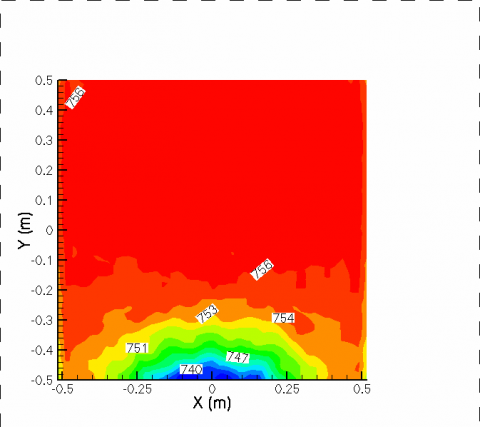(b) Upper surface of the cooking chamber

Figure 5. Temperature distribution at time t = 4.5 h

## figure_6.png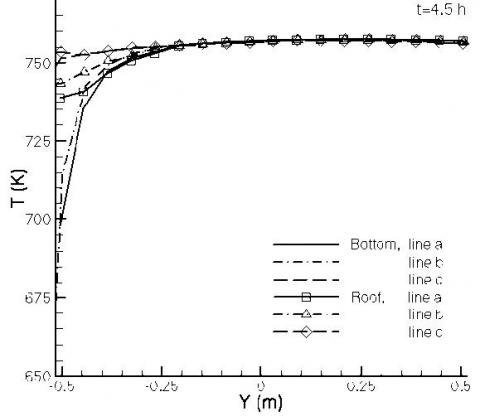Figure 6. Temperatures as a function of the Y coordinate on bottom and upper surfaces at time t = 4.5 h

## figure_7a.png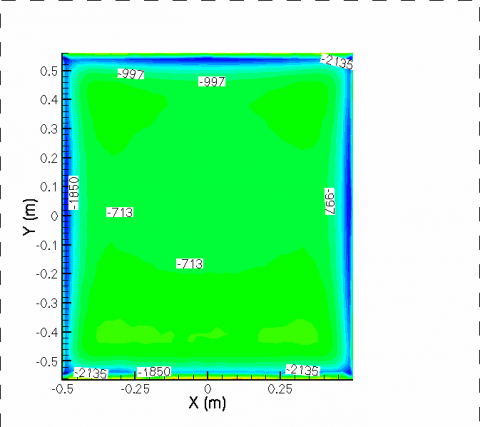(a) Total heat flux

## figure_7b.pngFigure 7. Heat fluxes distributions on the bottom surface of the cooking chamber, t = 1.3 h

Total heat flux comprises both radiative and convective heat flux and its value is negative because it enters the bottom surface.

This occurs because at that time, t = 1.3 h, the radiative heat transfer is mainly exchanged from the upper surface to the bottom one. In fact, observing Figure 7 b, the radiative heat flux plays the major role in heat exchange with radiative heat flux entering into the bottom surface.

Comparing Figure 7 a with Figure 7 b it is worth noting that the absolute value of the radiative heat transfer (which is negative) is larger than the total heat flux, since the convective heat transfer, which is quite low, is directed from the bottom surface toward the cold fluid entering the cooking chamber from the open section.

Different situation is observed in Figure 8 a for the same surface at the steady state, i.e. at t = 4.5 h. In fact, the total heat flux is positive, i.e. the flux is exiting the surface because the two opposite walls present nearly the same temperatures, thus the radiative heat flux is exchanged from the bottom surface to the lateral walls of the chamber.

In addition, the convective heat flux from the surface to the fluid now has a larger weight than before.

This observation is learned from Figure 8 b, where the radiative heat flux at the bottom surface is reported. In fact, it is positive except near the open surface because locally there is a large temperature difference between the upper and bottom surface locations, as Figure 6 eloquently displays. When the upper surface is considered, the heat flux distribution is quite different than that for the bottom surface.

## figure_8a.png(a) Total heat flux

## figure_8b.png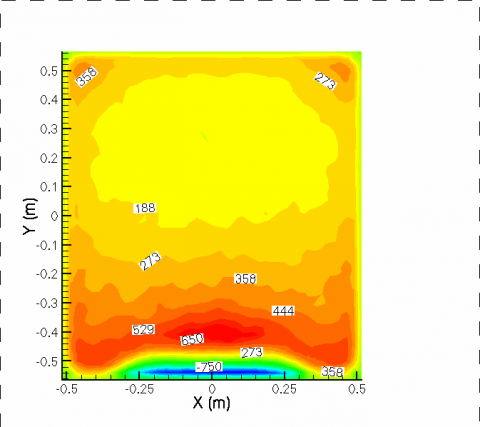Figure 8. Heat fluxes distribution on the bottom surface of the cooking chamber, t = 4.5 h

In fact, in Figure 9 a the total heat flux distribution shows very large values, about 6000 W/m2 at the central part of the surface at time t = 1.3 h. This is due to the large temperature difference existing between the upper and bottom surfaces, which induce huge radiative heat transfer, the main heat flux component.

This can be observed examining Figure 9 b, where the radiative component of heat flux is displayed. It can be observed that more than 95% of the total heat flux is supported by the radiative one, since the convective heat flux released from the surface toward the fluid is very weak on this surface.

Quite similar considerations can be gathered from Figure 10 a and Figure 10 b, where the total heat flux and the radiative component are displayed at time t = 4.5 h.

Local distribution of both fluxes is different than those during the heating phase, but observing the two distributions, the shapes are very similar, because as already said, the largest part of the heat flux is composed by the radiative component. Local values of the total heat flux are much lower than those during the heating phase, ranging from a maximum of  about 2700 W/m2,   bottom  surface,   to about 300 W/m2 in the inner part of the cooking chamber.

## figure_9a.png(a) Total heat flux

## figure_9.jpg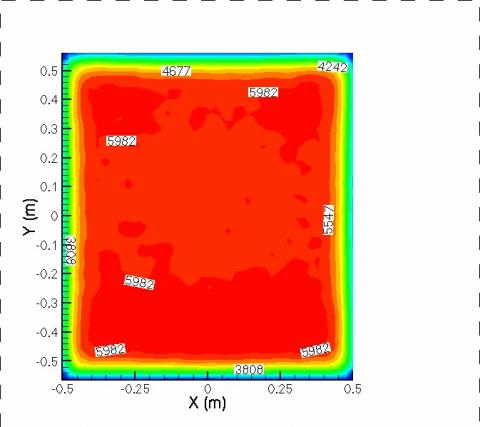Figure 9. Heat fluxes distribution on the upper surface of the cooking chamber, t = 1.3 h

In order to better assess what has been described in terms of heat flux distribution over the surfaces, Table 3 displays, for the upper and bottom surfaces at the two considered times, the average values over each surface of the total heat flux and the radiative component of the heat flux.

On the bottom surface, during the heating phase (t = 1.3 h) the radiative and convective heat flux component are opposed, the first entering the surface whereas the second exiting it.

Anyway its value is extremely weak. Instead, at t = 4.5 h (steady-state regime) the radiative heat flux accounts for no more than 75% of the total heat flux, thus the convective one plays a more important role during this regime. The situation is different examining the upper surface because in this case the radiative heat flux is always prevailing, accounting for more than 98% of the total heat flux.

Figure 11 displays the temperature distribution in the Y-Z plane for the whole domain at steady state condition, t = 4.5 h.

The distribution is nearly uniform inside the cooking chamber, delimited by black lines.

## figure_10a.png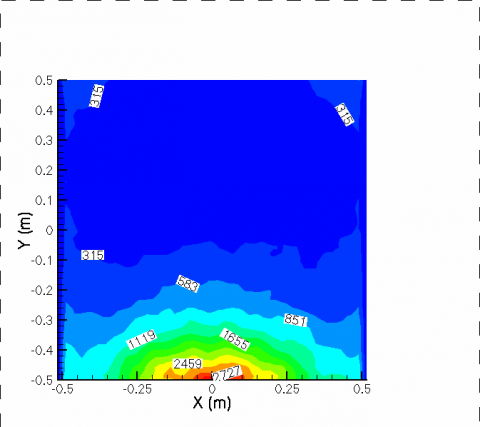(a) Total heat flux

## figure_10b.png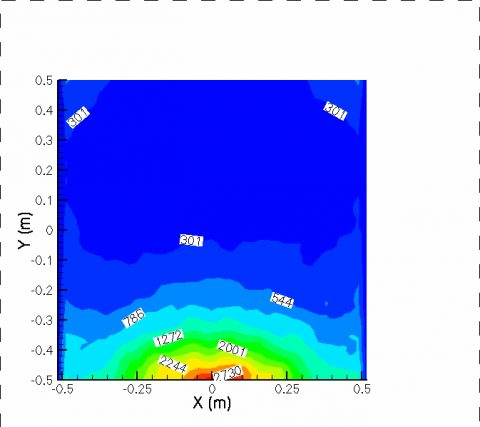Figure 10. Heat fluxes distribution on the upper surface of the cooking chamber, t = 4.5 h

Table 3. Total and radiative heat flux through the bottom and the upper surfaces of the cooking chamber during starting (t = 1.3 h) and steady state (t = 4.5 h) conditions

 Total Heat Flux (W. m-2) Radiative Heat Flux (W. m-2) t=1.3 h t=4.5 h t=1.3 h t=4.5 h Bottom -1,109.9 291.4 -1,241.1 215.5 Upper 5,282.2 579.6 5,237.6 570.7

## figure_11.png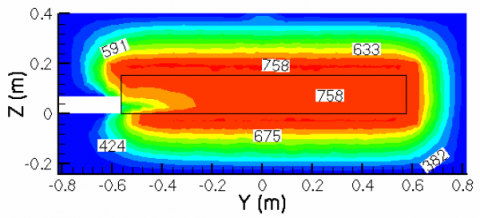Figure 11. Temperature distribution on plane Y-Z of the cooking chamber, t = 4.5 h

The two resistances show the same temperature value, whereas the rock wool represents a very good insulating material, since temperatures near the outside walls are very low. It is required for safety reasons that the temperature of the outside  shell   should  be  lower than 310 K,  and   the insulating thickness is able to maintain such temperature value. Figure 12 a and Figure 12 b show the velocity distribution in the Y-Z plane at the two times considered. It is to observe that the air enters the cooking chamber, showing a positive value of velocity, over the bottom surface.

It is clear that the flow enters over the bottom surface then, once the air is heated it goes upward and then flowing along the upper surface, exits the cooking chamber. The velocities are very low, with values around some cm/s.

## figure_12.png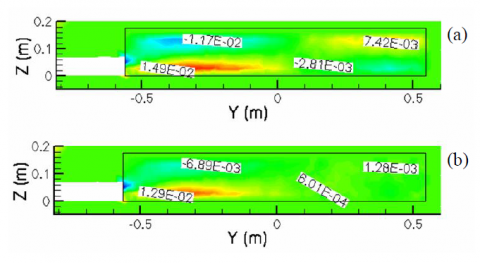Figure 12. Average velocity distribution on plane yz of the cooking chamber: (a) t = 1.3 h; (b) t = 4.5 h

5. Conclusions

The numerical analysis of an innovative electric oven for cooking pizzas has been accomplished. The thermal and fluid dynamic analysis evidences that the main heat transfer mechanism is radiation between the two main surfaces of the cooking chamber, upper and bottom. Anyway, the two surfaces have a different behavior. In fact the upper one is always characterized by radiative heat transfer which accounts for more than 95% of the total heat flux. Instead, on the bottom surface during the heating phase the prevalent component is the radiative one, whereas during the steady state regime the convective heat flux is about 25% of the total heat flux. This situation is unfavorable for cooking pizzas, since the convective heat transfer tends to cool pizzas in the oven. The thickness of the insulating material provides low temperature for the outside shell, as required for safety reasons.

Acknowledgment

The authors would like to thank the electrical oven manufacturer, Forni Izzo s.r.l., for providing useful technical data regarding design and operating conditions of the oven used for this work.

Nomenclature
 A surface, m2 c specific heat, J. kg-1. K-1 egen energy source term, W Fij configuration factor, - $\overline{g}$ gravitational acceleration, m. s-2 G Irradiance, W. m-2 K integral kernel, - J Radiosity, W. m-2 Nu local Nusselt number h Lref/l, - p pressure, Pa q heat flux, W. m-2 ri Position vector, m t time, s T temperature, K T0 operating temperature, K $\overline{\mathrm{V}}$ velocity, m. s-1 Greek symbols α thermal diffusivity, m2. s-1 β thermal expansion coefficient, K-1 ε emissivity, - λ thermal conductivity, W.m-1. K-1 ρ density kg. m-3 µ dynamic viscosity, kg. m-1.s-1 σ Stefan-Boltzmann constant, W. m-2. K-4 Subscripts eff effective i,j at location i, j p at constant pressure
References

 Ministero delle Politiche Agricole, Alimentari and Forestali, Disciplinare di produzione della specialità tradizionale garantita “Pizza Napoletana”, 2010, Gazzetta Ufficiale della Repubblica Italiana. (in Italian).

 P.A. Beauchemin and M. Tampier, Emissions from Wood-Fired Combustion Equipment, E.S. Inc. Editor. 2008: North Vancouver, B.C.

 U.S. Environmental Protection Agency, Emission Factor Documentation For AP-42 Section 1.6Wood Waste Combustion In Boilers. 1993 Research Triangle Park, NC: April, 1993.

 G.G. Choudhry and O. Hutzinger, “Mechanistic aspects of the thermal formation of halogenated organic compounds including polychlorinated dibenzo‐p‐dioxins,” Toxicol Environ Chem., vol. 5, no.  1, pp. 67-93, 1982. DOI: 10.1080/02772248209356985.

 T. Salthammer, H. Klipp, R. D. Peek and R. Marutzky. “Formation of polychlorinated dibenzo-p-dioxins (PCDD) and polychlorinated dibenzofurans (PCDF) during the combustion of impregnated wood,” Chemosphere, vol. 30, no. 11, pp. 2051-2060, 1995. DOI: 10.1016/0045-6535(95)00083-K.

 T. Salthammer, T. Schripp, S. Wientzek and M. Wensing, “Impact of operating wood-burning fireplace ovens on indoor air quality,” Chemosphere, vol. 103, pp. 205-211, 2014. DOI: 10.1016/j.chemosphere.2013.11.067.

 T. Salthammer. “Critical evaluation of approaches in setting indoor air quality guidelines and reference values,” Chemosphere, vol. 82, no. 11, pp. 1507-1517. DOI: 10.1016/j.chemosphere.2010.11.023.

 L. Gustavsson and M. L. Karlsson, Volatile Organic Compounds — Emissions from Biomass Combustion, in Advances in Thermochemical Biomass Conversion, A. V. Bridgwater, Editor. 1993, Springer Netherlands: Dordrecht, pp. 1522-1532.

 G. Buonanno, L. Morawska, L. Stabile and A. Viola. “Exposure to particle number, surface area and PM concentrations in pizzerias,” Atmos Environ, vol.  44, no. 32, pp. 3963-3969, 2010. DOI: 10.1016/j.atmosenv.2010.07.002.

 P. Verboven, N. Scheerlinck, J. De Baerdemaeker and B. M. Nicolaı, “Computational fluid dynamics modelling and validation of the isothermal airflow in a forced convection oven,” J Food Eng, vol. 43, no. 1, pp. 41-53, 2000. DOI: 10.1016/S0260-8774(99)00131-4.

 H. Mistry, S. Ganapathisubbua, S. Dey, P. Bishnoi and J. L. Castillo. “A methodology to model flow-thermals inside a domestic gas oven,” Appl Therm Eng, vol. 31, no. 1, pp. 103-111, 2011. DOI: 10.1016/j.applthermaleng.2010.08.022.

 M. Boulet, B. Marcos, M. Dostie and C. Moresoli. “CFD modeling of heat transfer and flow field in a bakery pilot oven,” J Food Eng, vol. 97, no. 3, pp. 393-402, 2010. DOI: 10.1016/j.jfoodeng.2009.10.034.

 S.N. Singh and D.K. Singh. “Study of combined free convection and surface radiation in closed cavities partially heated from below,” H&TECH, vol.33, no.2, pp.1-8, 2015. DOI: 10.18280/ijht.330201.

 S. Bouabdallah, D. Chati, B. Ghernaout, A. Atia and A. Laouirate. “Turbulent mixed convection in enclosure containing a circular/square heat source,” H&TECH, vol. 34, no. 3, pp.446-454, 2015. DOI: 10.18280/ijht.340314.

 H. K. Versteeg and W. Malalasekera, An Introduction to Computational Fluid Dynamics - The Finite Volume Method, 1995: Addison Wesley Longman Ltd.

 J. R. Howell, M. P. Menguc and R. Siegel, Thermal radiation heat transfer, CRC press, 2010.